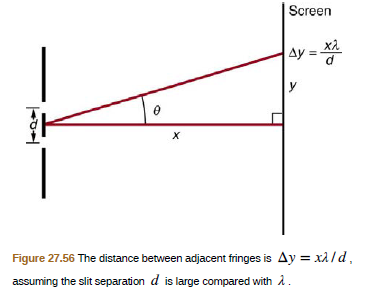Chapter 27, Problem 19PE

Chapter
Section
Textbook Problem

Using the result of the problem above, calculate the distance between fringes for 633-nm light falling on double slits separated by 0.0800 mm, located 3.00 m from a screen as in Figure 27.56.To determine

The distance between fringes for 633 nm light falling on double slits separated by 0.0800 mm, located 3 m from a screen as shown in figure 27.56.

Explanation

Given info:

Figure shows a double slit located a distance x, from a screen, with the distance from a screen, with the distance from the center of the screen given by y . When the distance d between the slits is relatively large, there will be numerous bright spots.

Formula used:

Δy=xλd

Calculation:

We have, x=3m, λ=633nm, d=0.0800mm.

Substituting these values in above, relation we get

Δλ=3

Still sussing out bartleby?

Check out a sample textbook solution.

See a sample solution

The Solution to Your Study Problems

Bartleby provides explanations to thousands of textbook problems written by our experts, many with advanced degrees!

Get Started

Find more solutions based on key concepts